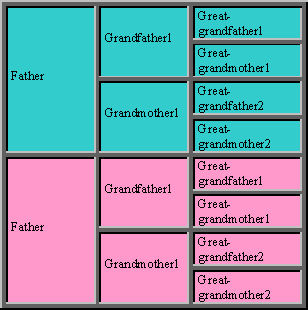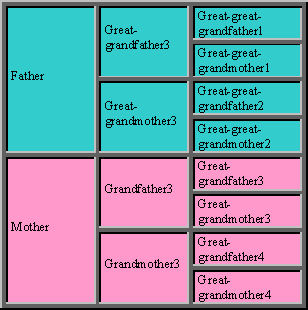# Braque du Bourbonnais

## Inbreeding calculation

• ### The path method:

• The simplest method to compute the Coefficient Of Inbreeding ("COI" or "F") is the path method. It consists in determining each of the possible paths from the father to the mother going trough a common ancestor. This will give all the possible paths for an allele to be given to your puppy at the same time by his father and his mother.

For that, we are going to use a classic representation of the pedigree in which the father and his ancestors are in blue and the mother and her ancestors are in pink:If none ancestor is at the same time in the blue part and in the pink part, there is no common ancestor, and the inbreeding is null. If an ancestor is present in the 2 parts of the pedigree, he is a common ancestor, so we have to determine each of the paths leading from the father to the mother and passing through him. For each of those path, we compute the depth (the number of dogs it contains) that we are going to call N.

Each path adds to the total inbreeding:
(1/2)^N = 0.5x0.5x... (N times 0.5)

Then, it is enough to find all the common ancestors, then each of the paths between the father, the common ancestor and the mother, to compute the inbreeding induced by each of those paths, and to add all of them.

Did you understand? Not quite ? Perhaps a few examples will help...

• ### A few examples of coefficient of inbreeding calculations:

• #### Brother/sister: 25%

• Here, you are going to marry the sire "P" to his sister "M", then doing inbreeding on the great parents "GP1" and "GM1".

Step by step explanation:

 1st step: Here is the pedigree• #### Father/sister - mother/son: 25%

• Here, we are going to marry the bitch "M" to her father, a common case when one has got a great champion (it is the same if you marry a great champion bitch to her son).

Step by step explanation:

 1st step: Here is the pedigree• #### Half-brother/half-sister: 12.5%

• It's a softer way to make inbreeding on a champion (here the common grand father "GP1").

Step by step explanation:

 1st step: Here is the pedigree• #### Uncle/niece - aunt/nevew: 12.5%

• Step by step explanation:

 1st step: Here is the pedigree• #### Cousin/cousin: 6.25%

• Step by step explanation:

 1st step: Here is the pedigree• ### Importance of the pedigree depth:

• Note that in the pedigree examples above, we have shown 3 generations only. We have implicitly made the hypothesis that none of the ancestors after 3 generations were related.

This hypothesis were useful to have simple computations, but the only mean to be sure that it is true would be to know all of those ancestors, which is impossible. So, when one compute an inbreeding coefficient, it is done on a given pedigree depth. The more you increase the depth of the pedigree, the more chances you have to find new common ancestors, and the more the inbreeding coefficient increases.

From that, we can conclude that the comparison of two inbreeding coefficients makes sense only if the depths of the two pedigrees are equal.

• ### Case of a common ancestor whose coefficient of inbreeding is not null:

• In the case where one of the common ancestors has a non null coefficient of inbreeding, one affects to each path passing through this ancestor the coefficient (1 + F) in the computation.

In the first example (brother-sister marriage), if we suppose that the grand-father "GP1" has already got an inbreeding coefficient of 10% (but the grand-mother GM1 0%), we obtain:

F= (1/2)^3*(0.1 + 1) + (1/2)^3 = 0,1375 + 0,125 = 0.2625 -> the coefficient of inbreeding is 26.25% instead of 25%